# Texas Go Math Grade 2 Lesson 4.4 Answer Key Identify Parts of a Whole

Refer to our Texas Go Math Grade 2 Answer Key Pdf to score good marks in the exams. Test yourself by practicing the problems from Texas Go Math Grade 2 Lesson 4.4 Answer Key Identify Parts of a Whole.

## Texas Go Math Grade 2 Lesson 4.4 Answer Key Identify Parts of a Whole

Explore

Circle the shapes that show equal parts. Name the equal parts shown.Explanation:
Each equal part of a whole is called one-half.
It is expressed as 1/2 and read as one over two or one upon two.
One eighth is one part of eight equal sections.
Two eighths is one quarter and four eighths is a half.

HOME CONNECTION • Your child completed this sorting activity with shapes to review the concept of equal parts.
Yes, know the child get the concept of equal parts of a whole.
The whole is divided in to two halves, one paper can be folded 2 halves,
that two halves folded again for fourths, and again folded for eighths.

Math Talk
Mathematical Processes

Explain how the two circles are different.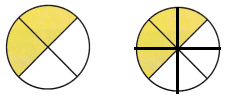Explanation:
Each equal part of a whole is called one-half.
One eighth is one part of eight equal sections.
Two eighths is one quarter and four eighths is a half.

Model and Draw

Can you name the shaded amount as some equal parts?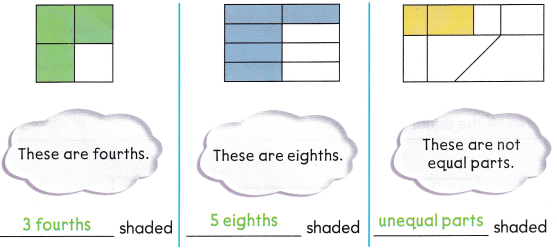Share and Show

If there are equal parts, write the amount shaded. If the parts are not equal, write unequal parts.

Question 1.Whole
Explanation:
When we divide a whole into equal parts, each part is a fraction of the whole.
So, the shaded part represents the whole.

Question 2.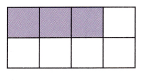Three eighths
Explanation:
If you divide something into 8 equal parts, each is one-eighth of the whole.
in the above rectangle 3-eighths are shaded.

Question 3.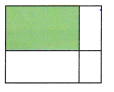Explanation:
In the above picture it is divided into un equal parts.
1 shaded part is also unequal.

Question 4.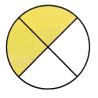Explanation:
If a whole is divided into four equal parts, each part is a FOURTH.
A fourth is obtained by dividing a whole (1) by 4.
In the above picture two fourths are shaded.

Problem Solving

If there are equal parts, write the amount shaded. If the parts are not equal, write unequal parts.

Question 5.Two un equal parts are shaded.
Explanation:
The above Hexagon is divided into unequal parts.

Question 6.Explanation:
The above picture is divided into eight equal parts.
Six eighths are shaded among it.

Question 7.

• Use yellow to shade 3 fourths of one shape.
• Use green to shade 7 eighths of one shape.
• Use blue to shade 2 halves of one shape.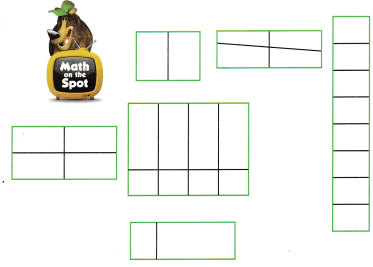Explanation:
The above given pictures which have equal parts are represented with different colors,
Yellow color represents to shade 3 fourths of one shape.
Green color represents to shade 7 eighths of one shape.
Blue color represents to shade 2 halves of one shape.

Question 8.
H.O.T. Does this shape show fourths? Explain.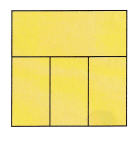Explanation:
As the given shape is not divided into equal parts.

Question 9.
Which shape is not divided into eighths?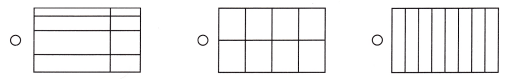Explanation:
The above picture option (A) is not divided into eighths.
If a whole is divided into eight equal parts, each part is an Eighth.
An eighth is obtained by dividing a whole (1) by 8.

Question 10.
Which shape is not divided into halves?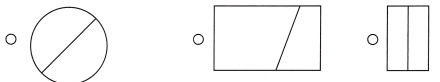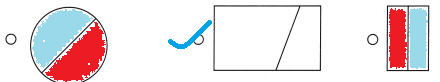Explanation:
The above picture option (B) is not divided into halves.
If a whole is divided into two equal parts, each part is a half.
A half is obtained by dividing a whole (1) by 2.

Question 11.
Which shape is not divided into fourths?Explanation:
The above picture option (A) is not divided into fourths.
If a whole is divided into four equal parts, each part is a fourth.
A fourth is obtained by dividing a whole (1) by 4.

Question 12.
TEXAS Test Prep Angelo drew this shape and shaded some of the parts. What amount is shaded?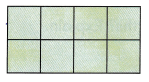(A) 1 eighth
(B) 1 fourth
(C) 8 eighths
Option (C)
Explanation:
The above picture option (C) is divided into eighths.
If a whole is divided into eight equal parts, each part is an Eighth.
An eighth is obtained by dividing a whole (1) by 8.
There were 8 equal parts, all parts are shaded.

TAKE HOME ACTIVITY • Ask your child to show how he or she solved an exercise in the lesson.
Child gets the concept of dividing the picture in to equal parts or not divided in to un equal parts.

### Texas Go Math Grade 2 Lesson 4.4 Homework and Practice Answer Key

If there are equal parts, write the amount shaded. If the parts are not equal, write unequal parts.

Question 1.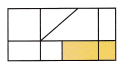Explanation:
The above picture is has unequal parts.

Question 2.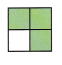Explanation:
The above picture divided into fourths.
If a whole is divided into four equal parts, each part is a fourth.
A fourth is obtained by dividing a whole (1) by 4.

Question 3.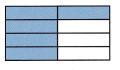Explanation:
The above picture is divided into eighths.
If a whole is divided into eight equal parts, each part is an Eighth.
An eighth is obtained by dividing a whole (1) by 8.

Question 4.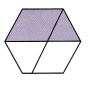Explanation:
The above picture is has unequal parts.

Problem Solving

Question 5.
Multi-Step

• Use yellow to shade 5 eighths of one shape.
• Use green to shade 2 halves of one shape.
• Use blue to shade 3 fourths of one shape.Explanation:
The above given pictures which have equal parts are represented with different colors,
yellow to shade 5 eighths of one shape.
green to shade 2 halves of one shape.
blue to shade 3 fourths of one shape.

Lesson Check

Question 6.
Andy drew this shape and shaded some of the parts. What amount is shaded?(A) 3 fourths
(B) 1 eighth
(C) 4 fourths
Option (C)
Explanation:
The above picture divided into fourths.
If a whole is divided into four equal parts, each part is a fourth.
A fourth is obtained by dividing a whole (1) by 4.

Question 7.
Alice baked a pie and cut it into equal slices. She gave 5 slices of the pie to her friends. Which pie is shaded to show how much she gave her friends?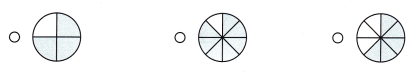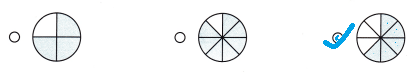Explanation:
The above pie divided into eighths.
If a whole is divided into eight equal parts, each part is an eighth.
An eighth is obtained by dividing a whole (1) by 8.
Out of 8 parts of pie slices, She gave 5 slices of the pie to her friends.
It is represented with 5 shaded parts.

Question 8.
Mark drew these shapes. Which shape shows 1 fourth shaded?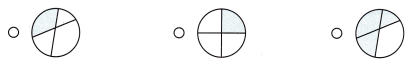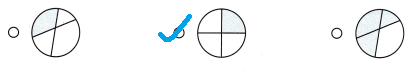Explanation:
The above picture divided into fourths.
If a whole is divided into four equal parts, each part is a fourth.
A fourth is obtained by dividing a whole (1) by 4.
Out of 4 parts of circle 1 fourth is shaded.

Question 9.
Jackie drew .this shape. What amount is shaded?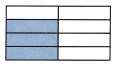(A) 3 eighths
(B) 5 eighths
(C) 2 eighths